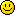[ moler2000 @ 14.06.2020. 06:33 ] @
 Pozdrav! Nedavno sam počeo da učim python. Na edX platformi radim kurs za početnike i došao sam do zadatka, koji nikako ne mogu da riješim. Naime, zadatak traži se zadani broj pretvori u binarni, bez upotrebe funkcije if i petlji. Pokušao sam da uradim zadatak uz upotrebu operatora za cjelobrojno dijeljenje i operatora za ostatak ali bezuspješno. Molio bih vas da mi objasnite na koji način mogu da riješim ovaj zadatak. Tekst zadatka glasi ovako: Code: number = 215 #You may modify the lines of code above, but don't move them! #When you Submit your code, we'll change these lines to #assign different values to the variables. # #The number above is given in base 10. Let's convert it to #base 2 and print it in binary. For example, 215 can be written #in binary as 11010111. # #Each digit of that string corresponds to a power of 2. The far #left digit represents 128, then 64, then 32, then 16, then 8, #then 4, then 2, and then finally 1 at the far right. # #To convert the number to binary, first check to see if it is #greater than or equal to 128. If it is, your first digit is 1. #If not, your first digit is 0. If the number was greater than #128, subtract 128 (because the 128 is captured by the first #digit of the string). # #Then, repeat that process for 64, 32, 16, 8, 4, 2, and 1. # #For example: # #215 is >= 128: 1 #87 is >= 64: 11 #23 is not >= 32: 110 #23 is >= 16: 1101 #7 is not >= 8: 11010 #7 is >= 4: 110101 #3 is >= 2: 1101011 #1 is >= 1: 11010111 # #Note that although we use 'if' a lot to describe this problem, #this can be done entirely with floor division and modulus. #Remember, if you convert a boolean to an integer, True becomes #1 and False becomes 0. # #Note that we always work with binary in 8-bit chunks: the #number 7 would be 00000111, not just 111. That's because inside #the computer, 8 1s and 0s make a byte, which is the smallest #practical unit of storage (rarely are bits used outside 8-bit #bytes). # #Print the string that results from this conversion. #Add your code here!
[ Deunan @ 14.06.2020. 11:36 ] @
Bez ikakve petlje i IF operatora?
Jedino "rucno" da ispises. Npr.:
Code:

num= 215
print(f"{num // 128}{num % 128 // 64}{num % 64 // 32}{num % 32 // 16}{num % 16 // 8}{num % 8 // 4}{num % 4 // 2}{num % 2 // 1}")
[ mjanjic @ 14.06.2020. 17:12 ] @
Očekuje se da je broj u opsegu 0-255, tj. da se uvek prikazuje sa 8 bita u binarnom brojnom sistemu: 00000000 - 11111111, tako da jedino to može da bude zabuna, ostalo je jasno. Može da se uradi posebna linija koda za svaki stepen broja 2, a može i u jednoj kao što je Deunan napisao, samo što nisam siguran da od početnika očekuju takvu "efikasnost".
[ moler2000 @ 15.06.2020. 07:37 ] @
Citat:
Deunan:
Bez ikakve petlje i IF operatora?
Jedino "rucno" da ispises. Npr.:
Code:

num= 215
print(f"{num // 128}{num % 128 // 64}{num % 64 // 32}{num % 32 // 16}{num % 16 // 8}{num % 8 // 4}{num % 4 // 2}{num % 2 // 1}")

Hvala na pomoći Deunan! Iz tvog rješenja sam naučio i šta radi f-string, pošto se sa ovakvim načinom ispisa još nisam susretao. Uglavnom, shvatio sam gdje sam napravio grešku, pa sam na osnovu tvog rješenja uradio zadatak u skladu sa mojim trenutnim znanjemCode:

number = 215

bin_num_0 = str(number // 128)
bin_num_1 = str(number % 128 // 64)
bin_num_2 = str(number % 64 // 32)
bin_num_3 = str(number % 32 // 16)
bin_num_4 = str(number % 16 // 8)
bin_num_5 = str(number % 8 // 4)
bin_num_6 = str(number % 4 // 2)
bin_num_7 = str(number % 2 // 1)

print (bin_num_0 + bin_num_1 + bin_num_2 + bin_num_3 + bin_num_4 + bin_num_5 + bin_num_6 + bin_num_7)